International
Tables for
Crystallography
Volume D
Physical properties of crystals
Edited by A. Authier

International Tables for Crystallography (2006). Vol. D, ch. 1.1, pp. 30-31

## Section 1.1.4.10.7.2. Independent components of symmetric axial tensors according to the following point groups

A. Authiera*

aInstitut de Minéralogie et de la Physique des Milieux Condensés, Bâtiment 7, 140 rue de Lourmel, 75015 Paris, France

#### 1.1.4.10.7.2. Independent components of symmetric axial tensors according to the following point groups

| top | pdf |

Some axial tensors are also symmetric. For instance, the optical rotatory power of a gyrotropic crystal in a given direction of direction cosines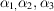is proportional to a quantity G defined by (see Section 1.6.5.4)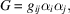where the gyration tensoris an axial tensor. This expression shows that only the symmetric part ofis relevant. This leads to a further reduction of the number of independent components:

• (i) Triclinic system

 (a) Group 1:(b) Group: all components are equal to zero.

• (ii) Monoclinic system

 (a) Group 2:(b) Group m:(c) Group: all components are equal to zero.

• (iii) Orthorhombic system

 (a) Group 222:(b) Group:(c) Group: all components are equal to zero.

• (iv) Trigonal, tetragonal and hexagonal systems

 (a) Groups 3, 32, 4, 42, 6, 62:(b) Group:(c) Group:(d) Groups,,,,,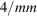,,and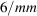: all components are equal to zero.

• (v) Cubic and spherical systems

 (a) Groups 23, 432 and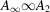:(b) Groups,,and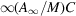: all components are equal to zero.

In practice, gyrotropic crystals are only found among the enantiomorphic groups: 1, 2, 222, 3, 32, 4, 422, 6, 622, 23, 432. Pasteur (1848a,b) was the first to establish the distinction between molecular dissymmetry' and crystalline dissymetry'.

### References

Pasteur, L. (1848a). Recherches sur les relations qui peuvent exister entre la forme cristalline, la composition chimique et le sens de la polarisation rotatoire. Ann. Chim. (Paris), 24, 442–459.Google Scholar
Pasteur, L. (1848b). Mémoire sur la relation entre la forme cristalline et la composition chimique, et sur la cause de la polarisation rotatoire. C. R. Acad Sci. 26, 535–538.Google Scholar